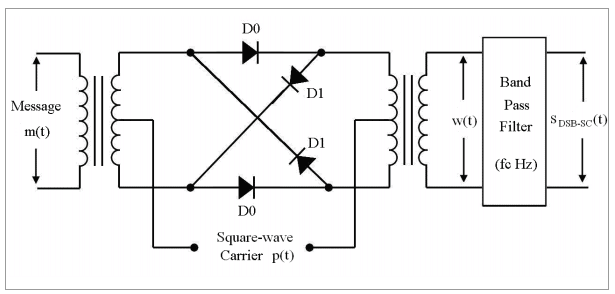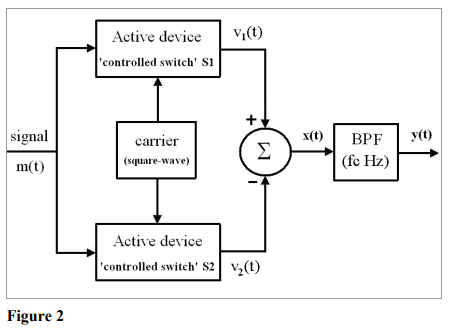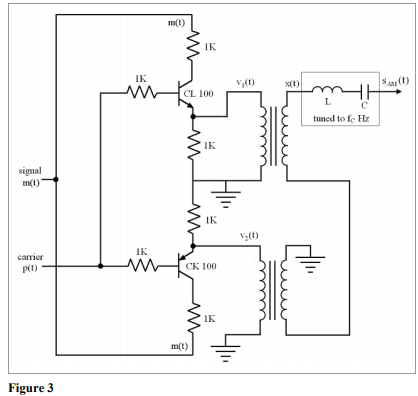Amplitude Modulation

# Amplitude Modulation - Electronics and Communication Engineering (ECE)

 1 Crore+ students have signed up on EduRev. Have you?

AMPLITUDE MODULATION:

In amplitude modulation, the instantaneous amplitude of a carrier wave is varied in accordance with the instantaneous amplitude of the modulating signal. Main advantages of AM are small bandwidth and simple transmitter and receiver designs. Amplitude modulation is implemented by mixing the carrier wave in a nonlinear device with the modulating signal.

This produces upper and lower sidebands, which are the sum and difference frequencies of the carrier wave and modulating signal.

The carrier signal is represented by

c(t) = A cos(wct)

The modulating signal is represented by

m(t) = B sin(wmt)

Then the final modulated signal is

[1 + m(t)] c(t)

= A [1 + m(t)] cos(wct)

= A [1 + B sin(wmt)] cos(wct)

= A cos(wct) + A m/2 (cos((wc+wm)t)) + A m/2 (cos((wc-wm)t))

Because of demodulation reasons, the magnitude of m(t) is always kept less than 1 and the frequency much smaller than that of the carrier signal.

The modulated signal has frequency components at frequencies wc, wc+wm and wc-wm.

DSBSC:

Double Sideband Suppressed Carrier Modulation In amplitude modulation the amplitude of a high-frequency carrier is varied indirect proportion to the low-frequency (baseband) message signal. The carrier is usually a sinusoidal waveform, that is,

c(t)=Ac cos(ωct+θc)

Or

c(t)=Ac sin(ωct+θc)

Where:

Ac is the unmodulated carrier amplitude

ωc is the unmodulated carrier angular frequency in radians/s;

ωc =2πfcθc is the unmodulated carrier phase, which we shall assume is zero.

The amplitude modulated carrier has the mathematical form

ΦDSB-SC(t)= A(t) cos(ωct)

Where:

A(t) is the instantaneous amplitude of the modulated carrier, and is a linear function of the message signal m(t). A(t) is also known as the envelope of the modulated signal For double-sideband suppressed carrier (DSB-SC) modulation the amplitude is related to the message as follows:

A(t)=Ac(t) m(t)

Consider a message signal with spectrum (Fourier transform) M(ω) which is band limited to 2πB as shown in Figure 1(b). The bandwidth of this signal is B Hz and ωc is chosen such that ωc >> 2πB. Applying the modulation theorem, the modulated Fourier transform is

A(t) cos(ωct)= m(t) cos(ωct) ⇔ ½( M(ω - ωc)+ M(ω + ωc))

GENERATION OF DSBSC:

The DSB-SC can be generated using either the balanced modulator or the 'ring-modulator‘. The balanced modulator uses two identical AM generators along with an adder. The two amplitude modulators have a common carrier with one of them modulating the input message , and the other modulating the inverted message . Generation of AM is not simple, and to have two AM generators with identical operating conditions is extremely difficult.

Hence, laboratory implementation of the DSB-SC is usually using the 'ring-modulator‘, shown in figure 1.Figure 1

Figure 1: The ring modulator used for the generation of the double-side-band-suppressedcarrier (DSB-SC)

This standard form of DSB-SC generation is the most preferred method of laboratory implementation. However, it cannot be used for the generation of the AM waveform.

he DSB-SC and the DSB forms of AM are closely related as; the DSB-SC with the addition of the carrier becomes the DSB, while the DSB with the carrier removed results in the DSB-SC form of modulation. Yet, existing methods of DSB cannot be used for the generation of the DSB-SC. Similarly the ring modulator cannot be used for the generation of the DSB. These two forms of modulation are generated using different methods. Our attempt in this work is to propose a single circuit capable of generating both the DSB-SC and the DSB forms of AM.

THE MODIFIED SWITCHING MODULATOR:

The block diagram of the 'modified switching modulator‘ given in figure 1, has all the blocks of the switching modulator, but with an additional active device. In this case, the active device has to be of three terminals to enable it being used as a 'controlled switch‘. Another significant change is that of the ‗adder‘ being shifted after the active device. These www.VidyarthiPlus.in www.VidyarthiPlus.in changes in the 'switching-modulator‘ enable the carrier to independently control the switching action of the active device, and thus eliminate the restriction existing in the usual 'switching-modulator‘ (equation (2)). In addition, the same circuit can generate the DSBSC waveform. Thus the task of modulators given in figures 1 and 2 is accomplished by the single modulator of figure 3.Figure 2: The modified 'switching modulator‘ It is possible to obtain AM or the DSB-SC waveform from the 'modified switchingmodulator‘ of figure 3, by just varying, the amplitude of the square wave carrier . It may be noted that the carrier performs two tasks: (i) control the switching action of the active devices and (ii) control the depth of modulation of the generated AM waveform. Thus, the proposed modification in the switching modulator, enables the generation of both the AM and the DSB-SC from a single circuit. Also, it may be noted that the method is devoid of any assumptions or stringent difficult to maintain operating conditions, as in existing low power generation of the AM. We now implement the 'modified switching modulator‘ and record the observed output in the next Section.

Experimental results

The circuit implemented for testing the proposed method is given in figure 4, which uses transistors CL-100 and CK-100 for controlled switches, two transformers for the adder, followed by a passive BPF. The square-wave carrier and the sinusoidal message are www.VidyarthiPlus.in www.VidyarthiPlus.in given from a function generator (6MHz Aplab FG6M).The waveforms are observed on the mixed signal oscilloscope (100MHz Agilent 54622D, capable of recording the output in '.tif‘ format).Figure 3: The implementation of the modified 'switching modulator‘ to generate the AM and the DSB-SC waveform.

The modified switching modulator is tested using a single tone message of 706 Hz, with a square-wave carrier of frequency 7.78 KHz. The depth of modulation of the generated waveform can be varied either by varying the amplitude of the carrier or by varying the amplitude of the signal. Figure 5 has the results of the modulated waveforms obtained using the 'modified switching modulator‘. It can be seen that the same circuit is able to generate AM for varying depths of modulation, including the over-modulation and the DSB-SC. The quality of the modulated waveforms is comparable to that obtained using industry standard communication modules (like the LabVolt for example).

Properties of DSB-SC Modulation:

(a) There is a 180 phase reversal at the point where +A(t)=+m(t) goes negative. This is typical of DSB-SC modulation.

(b) The bandwidth of the DSB-SC signal is double that of the message signal, that is, BWDSB-SC =2B (Hz).

(c) The modulated signal is centered at the carrier frequency ωc with two identical sidebands (double-sideband) – the lower sideband (LSB) and the upper sideband (USB). Being identical, they both convey the same message component.

(d) The spectrum contains no isolated carrier. Thus the name suppressed carrier.

(e)The 180 phase reversal causes the positive (or negative) side of the envelope to have a shape different from that of the message signal. This is known as envelope distortion, which is typical of DSBSC modulation.

(f) The power in the modulated signal is contained in all four sidebands.

The document Amplitude Modulation - Electronics and Communication Engineering (ECE) is a part of Electronics and Communication Engineering (ECE) category.
All you need of Electronics and Communication Engineering (ECE) at this link: Electronics and Communication Engineering (ECE)

Track your progress, build streaks, highlight & save important lessons and more!(Scan QR code)

,

,

,

,

,

,

,

,

,

,

,

,

,

,

,

,

,

,

,

,

,

;